# Z-score on a sample set

In the last blog about Z-score, we talked about the odd of having a sample data point x from a given Normal distribution.

Today, we are not taking only 1 data point, but a set of n data points. We call the mean value of this set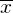, the question is: How likely do we have a sample set with size n whose mean is at least as extreme as?

Let’s return to the example of IQ-tests. Humans’ IQ is normally distributed with mean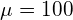and std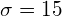(which means variance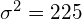). This time, says you are not taking the test alone, but with all your classmates. Your class has 20 students, and their average result on IQ-test is 106. The question is: how is your class’s IQ compared to other groups of 20 people?

Remember that in the original version of this example, you are the only one to take the IQ-test, and we compare your result to the full population on Earth. In this modified version, we change the size, from only you (size = 1) to all your class (size = 20).

In fact, we can map the current problem to the same state as the original problem, because the average value of a group of size n (n > 1) also follows Normal distribution, called Normal distribution of the mean. What we need is the mean and std of this distribution, let’s call them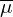and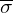, respectively.

Compute: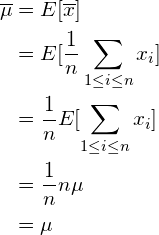Before computing, let’s revise some properties of Variance:

•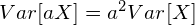,
•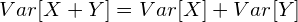if X and Y are independent.

Ok, let’s continue: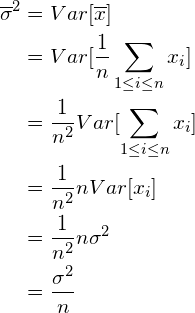So: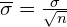(It worths mentioning thathas its own name, called the standard error of the sample.)

Well done! Now we know that:

The distribution of sample mean is a normal distribution with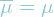and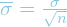.

To get the z-score of your class’s IQ, we should use this distribution. Do you remember the formula of Z-score? Apply it here:

Z-score =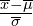or we can say in general:

Z-score =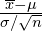For our case,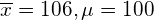and. Thus,

Z-score[class IQ] =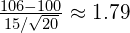Look it up on the z-table gets us a percentile of 96.3%, which means your class is in top 3.7% of the world (compared to any other groups of 20 people), that’s impressive!

Conclusion

In this blog, we introduced the distribution of sample mean, which is also a normal distribution with the same mean (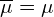) but different standard deviation (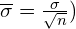.

## 4 thoughts on “Z-score on a sample set”

1.tdang says:

Very clear explanation. Any recommend about size of sample to get its mean close to normal distribution?

1.Tung.M.Phung says:

Thanks for your question. Let me try solving it!

First, we reformulate the problem:
You have:

• a normal distribution (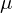and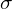are known).
• a generator that can generate data samples (you believe that this generator generates data following the above normal distribution).

You define: a confidence level and a confidence interval.
You ask for: the smallest sample size such that with the confidence level, the sample mean will fall into the confidence interval.

Seems complicated. Let’s make it concrete by putting real numbers on:
You have a normal distribution N(10, 2).
Suppose your generator also output values following N(10, 2).
Suppose “close” means the actual mean and the sample mean is not different for more than 0.1(i.e. 0.2).
You ask for the smallest sample size n, so that 95% of the random samples of size n have their means fall into the range [9.8, 10.2].

Solve:
For a 2-tailed z-test with 95% confidence level, the |z-score| needed is 1.96.
Substitute all those values to the equation Z-score =We have n to be 384.16. Thus, this is the smallest value of n needed to satisfy the above requirements.

Bests,

1.tdang says:

Well, I should have asked in a more specific way.
My question is on cases when we do not know exact information about the population (because if we already know about mean/std of the population, it seems getting stats on its sample is an unnecessarily).
In these cases, we can conduct a survey/measurement of a sample then can infer its stats to population. Then, a question raised: What size of sample should be?

Regards,
Truong Dang.

1.Tung.M.Phung says:

Thanks for the details. Actually, I was not sure if my answer matched your intended question.
However, the example above seems still come in handy.

2. A normal distribution’s signature is itsand. Thus, if we want our sample to match the population distribution, it is sufficient to haveandof the sample close to that of the population.
3. As a rule-of-thumb to determine whether a Z-test or a T-test is suitable for testing a hypothesis, the sample’s standard deviation (often denoted as s) is considered to be a good representative of the population’sif the sample size > 30.
4. The only problem is how to have the sample mean close to the population mean. On the example above, to compute the value of n, we actually did not assume anything about the mean of the population, we only assumed that the population’sis 2. The formula is: Z-score =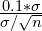, which gave us the needed value of the sample size is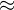384.
5. In conclusion, with a sample size of384, we have both the mean and standard deviation of the sample “close” to the population. Thus, a sample of this size can well represent the whole population.